# Average – Ignore Errors in Excel & Google Sheets

This tutorial will demonstrate how to calculate the average of a set of numbers, excluding error values, in Excel and Google Sheets.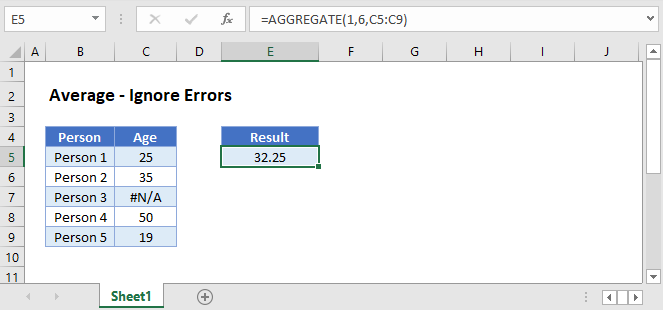## Error Issues With the AVERAGE Function

The AVERAGE Function calculates the mathematical mean of a set of numbers. However, when the input range includes an error value, the AVERAGE Function will output an error.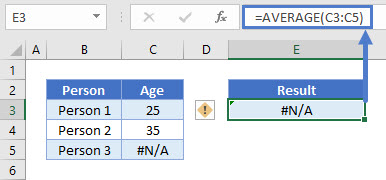In some cases, this may be the desired result. An error value can alert you to problems with your data. In other cases, it’s fine for your data to include error values, but you want to average the rest of the data.

To ignore cells with errors, we can use the AGGREGATE or AVERAGEIF functions.

## Ignore Errors With AGGREGATE Function

To ignore cells with error values use Excel’s AGGREGATE Function:

``=AGGREGATE(1,6,C3:C5)``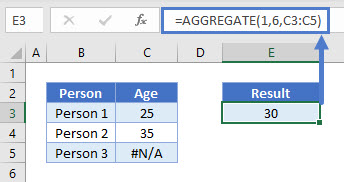The AGGREGATE Function allows you to use any of several mathematical summary functions, including AVERAGE, but with additional options on how the input data is processed. In this example, we utilize the option to ignore inputs with errors.

``=AGGREGATE(1,6,C3:C5)``

The Function requires 3 inputs

1. function number – This is the calculation to perform. Use 1 for AVERAGE.
2. options – To ignore error values in inputs, we use option 6.
3. input range – The range to calculate.

## Ignore Errors With AVERAGEIF Function

The AVERAGEIF Function can also be used to ensure that only specific numerical values are used in the calculation. Here we use “>0” to average only numbers greater than zero. This also eliminates any errors.

``=AVERAGEIF(B4:D4,">0")``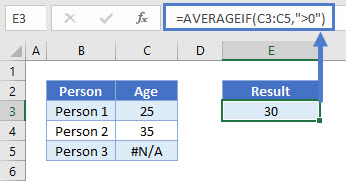## Ignore Errors With AVERAGE Function in Google Sheets

The AVERAGEIFS Function works in exactly the same way in Google Sheets as in Excel. However, the AGGREGATE Function is not available in Google Sheets.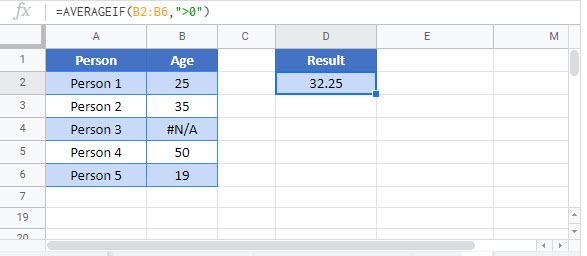### Excel Practice Worksheet

Practice Excel functions and formulas with our 100% free practice worksheets!